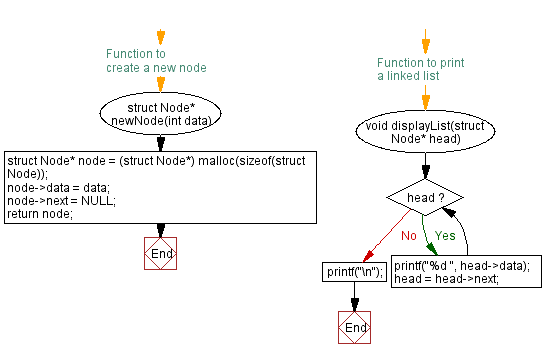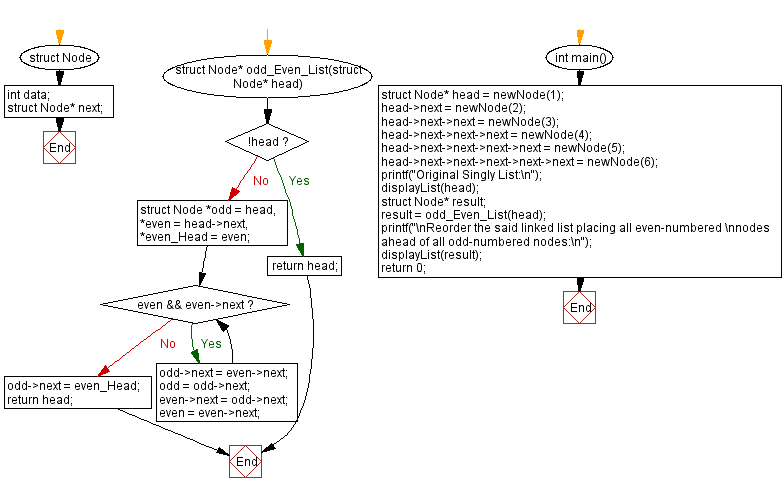﻿ C Program: Reorder even-numbered before odd in a singly linked list

# C Exercises: Reorder even-numbered before odd in a singly linked list

## C Singly Linked List : Exercise-30 with Solution

Write a C program to create and reorder a linked list placing all even-numbered nodes ahead of all odd-numbered nodes.

Sample Solution:

C Code:

``````#include<stdio.h>
#include <stdlib.h>

struct Node {
int data;
struct Node* next;
};

// Function to create a new node
struct Node* newNode(int data) {
struct Node* node = (struct Node*) malloc(sizeof(struct Node));
node->data = data;
node->next = NULL;
return node;
}

struct Node* odd_Even_List(struct Node* head) {
while (even && even->next) {
odd->next = even->next;
odd = odd->next;
even->next = odd->next;
even = even->next;
}
}

// Function to print a linked list
}
printf("\n");
}

int main() {
printf("Original Singly List:\n");
struct Node* result;
printf("\nReorder the said linked list placing all even-numbered \nnodes ahead of all odd-numbered nodes:\n");
displayList(result);
return 0;
}
```
```

Sample Output:

```Original Singly List:
1 2 3 4 5 6

Reorder the said linked list placing all even-numbered
nodes ahead of all odd-numbered nodes:
1 3 5 2 4 6
```

Flowchart :C Programming Code Editor:

What is the difficulty level of this exercise?

﻿